# GMAT Math : Calculating whether lines are parallel

## Example Questions

### Example Question #1 : Parallel Lines

Which of the following pairs of lines are parallel?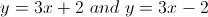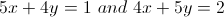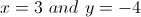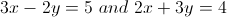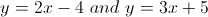Explanation:

Two lines are parallel if they have the same slope.  Let's go through the answer choices.

1.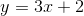and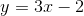: Both slopes are 3, parallel.

2.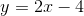and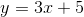: Slopes are 2 and 3, not parallel.

3.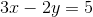and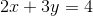: We need to put these two equations into the form ofto find the slope,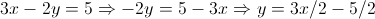, so the first slope is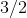.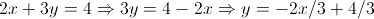, so the second slope is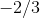.  These lines are perpendicular, not parallel.

4.and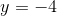: Slopes are undefined and 0, respectively, so not parallel.

5.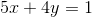and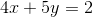: Let's again put these into the form of.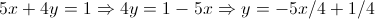, so the first slope is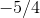.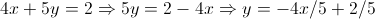, so the second slope is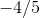.  The lines are not parallel.

### Example Question #2 : Calculating Whether Lines Are Parallel

Determine whether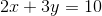and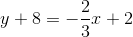are parallel lines.

No, because the slopes are not negative reciprocals of each other.

Yes, because the slopes are the same.

No, because the slopes are not similar.

Yes, because the slopes are negative reciprocals of each other.

Yes, because the slopes are the same.

Explanation:

Parallel lines have the same slope. Therefore, we need to find the slope once both equations are in slope intercept form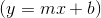: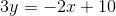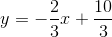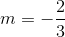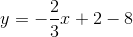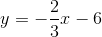The lines are parallel because the slopes are the same.

### Example Question #1 : Calculating Whether Lines Are Parallel

Three lines - one green, one blue, one red - are drawn on the coordinate axes. They have the following characteristics:

The green line has-intercept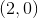and-intercept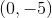.

The blue line has-interceptand-intercept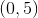.

The red line has-intercept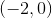and-intercept.

Which of these lines are parallel to each other?

Only the blue line and the red line are parallel to each other.

Only the green line and the red line are parallel to each other.

No two of these lines are parallel to each other.

Only the blue line and the green line are parallel to each other.

All three lines are parallel to one another.

Only the blue line and the red line are parallel to each other.

Explanation:

Use the intercepts to find the slopes of the lines.

Green: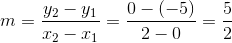Blue: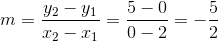Red: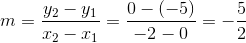The blue and red lines have the same slope; the green line has a different slope from the other two. That means only the blue and red lines are parallel.

### Example Question #4 : Calculating Whether Lines Are Parallel

What is the slope of a line that is parallel to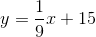?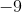None of the above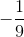Explanation:

Two lines are parallel if their slopes have the same value. Sincehas a slope of, any line parallel to it must also have a slope of### Example Question #5 : Calculating Whether Lines Are Parallel

Which of the following lines is parallel to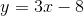?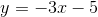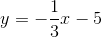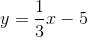Two of the answers provided are correct.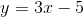Explanation:

Two lines are parallel to each other if their slopes have the same value. Since the slope ofis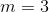,is the only other line provided that has the same slope.

### Example Question #6 : Calculating Whether Lines Are Parallel

Determine whether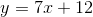and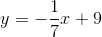are parallel lines.

No, because the slopes are not the same.

Cannot determine.

Yes, because the slopes are negative reciprocals of each other.

Yes, because the slopes are the same.

No, because the slopes are not negative reciprocals of each other.

No, because the slopes are not the same.

Explanation:

By definition, lines that are parallel to each other must have the same slope.has a slope of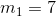andhas a slope of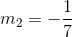, therefore they are not parallel because their slopes are not the same.

### Example Question #7 : Calculating Whether Lines Are Parallel

Tell whether the lines described by the following are parallel.

g(x) is a linear equation which passes through the points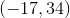and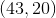.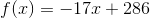They are not parallel, because they have different slopes.

There are not parallel, they are the same line.

They are parallel, because they have the same slope.

They are parallel, because their slopes are opposite reciprocals of each other.

They are not parallel, because they have different slopes.

Explanation:

Tell whether the lines described by the following are parallel.

g(x) is a linear equation which passes through the pointsandBegin by recalling that lines are parallel if their slopes are the same and they have different y-intercepts.

Let's find the slope of g(x)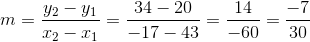Note that this slope is not the same as f(x), so we do not have parallel lines!

Tired of practice problems?

Try live online GMAT prep today.